src
re2c::Range Class Reference

`#include <range.h>`

Collaboration diagram for re2c::Range:[legend]

## Public Member Functions

~Range ()

Rangenext () const

uint32_t lower () const

uint32_t upper () const

## Static Public Member Functions

static Rangesym (uint32_t c)

static Rangeran (uint32_t l, uint32_t u)

static Rangeadd (const Range *r1, const Range *r2)

static Rangesub (const Range *r1, const Range *r2)

## Static Public Attributes

static free_list< Range * > vFreeList

## Friends

template<uint8_t >
Rangere2c_test::range (uint32_t n)

## Detailed Description

Definition at line 15 of file range.h.

## Constructor & Destructor Documentation

 re2c::Range::~Range ( )
inline

Definition at line 35 of file range.h.

36  {
37  vFreeList.erase (this);
38  }
static free_list< Range * > vFreeList
Definition: range.h:18

Here is the call graph for this function: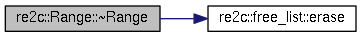## Member Function Documentation

 Range * re2c::Range::add ( const Range * r1, const Range * r2 )
static

Definition at line 26 of file range.cc.

27 {
28  Range * head = NULL;
29  Range * tail = NULL;
30  for (; r1 && r2;)
31  {
32  if (r1->lb < r2->lb)
33  {
35  r1 = r1->nx;
36  }
37  else
38  {
40  r2 = r2->nx;
41  }
42  }
43  for (; r1; r1 = r1->nx)
44  {
46  }
47  for (; r2; r2 = r2->nx)
48  {
50  }
52 }

Here is the caller graph for this function: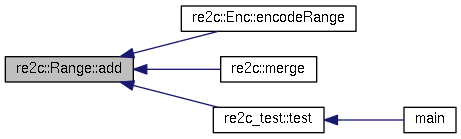uint32_t re2c::Range::lower ( ) const
inline

Definition at line 40 of file range.h.

40 { return lb; }

Here is the caller graph for this function: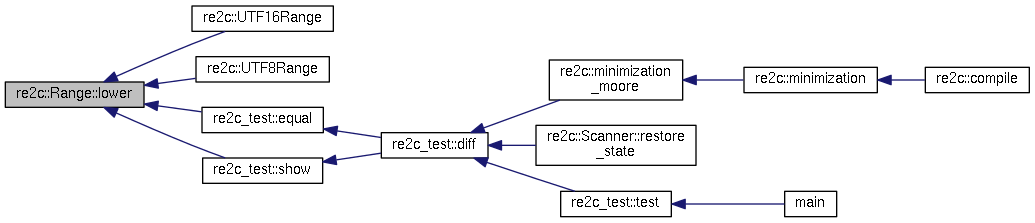Range* re2c::Range::next ( ) const
inline

Definition at line 39 of file range.h.

39 { return nx; }

Here is the caller graph for this function: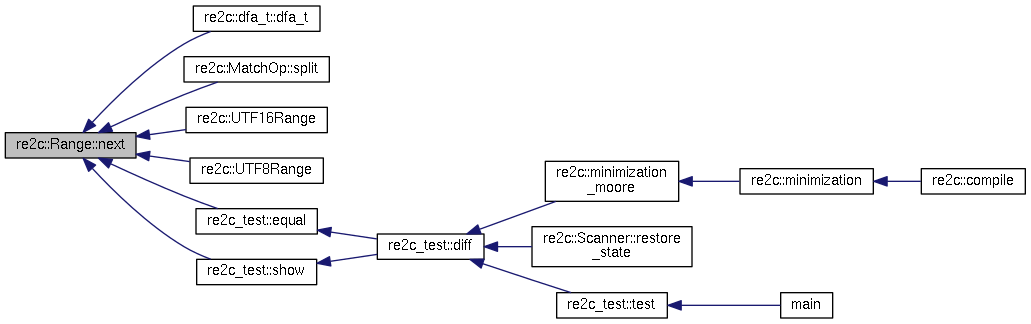static Range* re2c::Range::ran ( uint32_t l, uint32_t u )
inlinestatic

Definition at line 31 of file range.h.

32  {
33  return new Range (NULL, l, u);
34  }

Here is the caller graph for this function: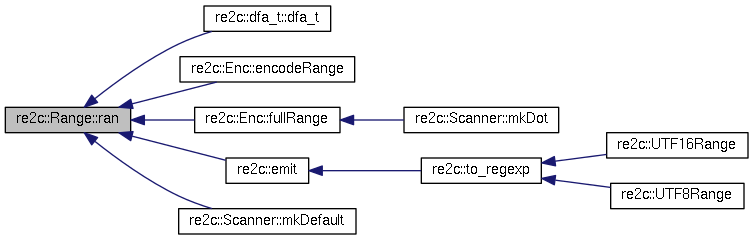Range * re2c::Range::sub ( const Range * r1, const Range * r2 )
static

Definition at line 61 of file range.cc.

62 {
63  Range * head = NULL;
64  Range ** ptail = &head;
65  while (r1)
66  {
67  if (!r2 || r2->lb >= r1->ub)
68  {
69  append (ptail, r1->lb, r1->ub);
70  r1 = r1->nx;
71  }
72  else if (r2->ub <= r1->lb)
73  {
74  r2 = r2->nx;
75  }
76  else
77  {
78  if (r1->lb < r2->lb)
79  {
80  append (ptail, r1->lb, r2->lb);
81  }
82  while (r2 && r2->ub < r1->ub)
83  {
84  const uint32_t lb = r2->ub;
85  r2 = r2->nx;
86  const uint32_t ub = r2 && r2->lb < r1->ub
87  ? r2->lb
88  : r1->ub;
89  append (ptail, lb, ub);
90  }
91  r1 = r1->nx;
92  }
93  }
95 }

Here is the caller graph for this function:static Range* re2c::Range::sym ( uint32_t c )
inlinestatic

Definition at line 27 of file range.h.

28  {
29  return new Range (NULL, c, c + 1);
30  }

Here is the caller graph for this function:uint32_t re2c::Range::upper ( ) const
inline

Definition at line 41 of file range.h.

41 { return ub; }

Here is the caller graph for this function:## Friends And Related Function Documentation

template<uint8_t >
 Range* re2c_test::range ( uint32_t n )
friend

## Member Data Documentation

 free_list< Range * > re2c::Range::vFreeList
static

Definition at line 18 of file range.h.

The documentation for this class was generated from the following files: